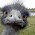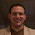### Big, Big Formula!

1) What does the following formula generate?
2) What does the answer to 1) have to do with the "analyticity" of mathematical knowledge?

(k+2){1 – [wz+h+j–q]2 – [(gk+2g+k+1)(h+j)+h–z]2 – [2n+p+q+z–e]2 – [16(k+1)3(k+2)(n+1)2+1–f2]2 – [e3(e+2)(a+1)2+1–o2]2 – [(a2–1)y2+1–x2]2 – [16r2y4(a2–1)+1–u2]2 – [((a+u2(u2–a))2 –1)(n+4dy)2 + 1 – (x+cu)2]2 – [n+l+v–y]2 – [(a2–1)l2+1–m2]2 – [ai+k+1–l–i]2 – [p+l(a–n–1)+b(2an+2a–n2–2n–2)–m]2 – [q+y(a–p–1)+s(2ap+2a–p2–2p–2)–x]2 – [z+pl(a–p)+t(2ap–p2–1)–pm]2}

1.english bob12:22 PM

Prime numbers?

2.Yes, the positive values of the polynomial are all prime.

Now, for question two.

3.e.b.: How the hell did you know?

Gene: And here I thought it had been proven that no polynomial could generate only primes. Does that apply only to polynomials of one variable? Has it not been proven? Where did you get the formula?

Question two: I don't yet see how it does.

4.Maybe it shows that there is regularity in even something seemingly unpredictable as prime numbers?

By the way, the answer to the first question can be googled by entering the formula into google ;)

5.Wa-a-a-ait a dog-bone minute! How the %@!*&\$\$%* can that thing generate only--or even any--primes when its initial parsing yields two factors (the first being k+2)?

6.Aha! I see: the missing datum in Gene's presentation of the formula is the proviso that the result be positive.

7.scineram6:42 PM

This.

8.Wabulon, I think you get it now -- it's only the positive values that are prime (as I mentioned!) and in the ccase the factorization becomes (k + 2) * 1.

9.Okay, but what about question two? What does this have to do with the analyticicationness of mathematical knowledge?

10."Okay, but what about question two? What does this have to do with the analyticicationness of mathematical knowledge?"

Hmmm... makes a mockery of the concept? Does anyone really want to argue that this equation was "contained in" the definition of a prime number? No, says I: this was a discovery, like, say, the discovery of DNA.

11.Oh, you were referring to the analytic/synthetic distinction. I wasn't sure if you meant "analytic" in the sense of "analytic function" or "analytic philosophy".

Because I'm an idiot, troll, know-it-all, etc.

12.Gene -

Quite right, positiveness was contained in your presentation all along.

I think you and perhaps S.B. miss the point on analyticity: Only God is the judge, we're just philosophers; He knows when it's there to know.

13.Andy Stedman2:13 PM

How do you know?

14."Because I'm an idiot, troll, know-it-all, etc."

It;s nice when you can have your own conversations, isn't it?